30 19 1K (1 Today)
Published: October 18, 2014
© 2014 - 2019 eriban
My first fractal flame. A tesselated impossible triangle. Very Escher like.

Credits
This fractal is heavily based on the Impossible fractal created by  which I really liked. I had one suggestion though how it could be further improved. Before I knew it, tatasz tricked me into taking this task upon myself by claiming it could not be done. This required me refreshing my knowledge on fractals and learning to use Apophysis, both very fun. The resulting image resembles the original by tatasz closely. The main difference is that the layout of the triangles is now such that edges of the same color do not touch anywhere. Despite the similarities of the resulting fractals, the sources are quite different. I recreated the fractal from scratch and used a different approach. For example, this fractal is created using 25 transforms (9 basic shapes, and 16 subsequent transformations) whereas the original used 32 transforms (27 basic shapes and 5 subsequent transformations). Anyway, thanks to tatasz for inspiring me to create fractal flames. This certainly won't be my last!

Source
<flame name="Triangles-v23" version="Apophysis 7x Version 15B" size="800 600" center="0 0" scale="320" angle="-0.523598775598299" rotate="30" oversample="2" filter="0.02" quality="1" background="0 0 0" brightness="1" gamma="30" estimator_radius="9" estimator_minimum="0" estimator_curve="0.2" enable_de="0" plugins="" >
<xform weight="9" color="0.5" symmetry="-1" square="1" coefs="1 0 0 1 0 0" post="9 0 -0.5 0.866025 0.25 2.74241" chaos="0 0 0 0 0 0 0 0 0 1 1 1 0 0 0 0 0 0 0 0 0 0 0 0 " opacity="0" name="square 1"/>
<xform weight="2" color="0.5" symmetry="-1" square="1" coefs="1 0 0 1 0 0" post="1 0 -1 1.732051 -3 1.44338" chaos="0 0 0 0 0 0 0 0 0 1 1 1 0 0 0 0 0 0 0 0 0 0 0 0 " opacity="0" name="square 2"/>
<xform weight="2" color="0.5" symmetry="-1" square="1" coefs="1 0 0 1 0 0" post="1 0 -1 1.732051 1 1.44338" chaos="0 0 0 0 0 0 0 0 0 1 1 1 0 0 0 0 0 0 0 0 0 0 0 0 " opacity="0" name="square 3"/>
<xform weight="2" color="0.5" symmetry="-1" square="1" coefs="1 0 0 1 0 0" post="1 0 -1 1.732051 -1 -2.020726" chaos="0 0 0 0 0 0 0 0 0 1 1 1 0 0 0 0 0 0 0 0 0 0 0 0 " opacity="0" name="square 4"/>
<xform weight="2" color="0.5" symmetry="-1" square="1" coefs="1 0 0 1 0 0" post="2 0 -0.5 0.866025 0.75 -0.721688" chaos="0 0 0 0 0 0 0 0 0 1 1 1 0 0 0 0 0 0 0 0 0 0 0 0 " opacity="0" name="square 5"/>
<xform weight="0.5" color="0.5" symmetry="-1" starblur="1" coefs="1 0 0 1 0 0" post="0.576 0 0 0.576 1.5 0.288675" starblur_power="3" starblur_range="0.5" chaos="0 0 0 0 0 0 0 0 0 1 1 1 0 0 0 0 0 0 0 0 0 0 0 0 " opacity="0" name="triangle 1"/>
<xform weight="0.5" color="0.5" symmetry="-1" starblur="1" coefs="1 0 0 1 0 0" post="0.576 0 0 0.576 -2.5 0.288675" starblur_power="3" starblur_range="0.5" chaos="0 0 0 0 0 0 0 0 0 1 1 1 0 0 0 0 0 0 0 0 0 0 0 0 " opacity="0" name="triangle 2"/>
<xform weight="0.5" color="0.5" symmetry="-1" starblur="1" coefs="1 0 0 1 0 0" post="0.576 0 0 0.576 -0.5 -3.17543" starblur_power="3" starblur_range="0.5" chaos="0 0 0 0 0 0 0 0 0 1 1 1 0 0 0 0 0 0 0 0 0 0 0 0 " opacity="0" name="triangle 3"/>
<xform weight="0.5" color="0.5" symmetry="-1" starblur="1" coefs="1 0 0 1 0 0" post="0.288 -0.498831 0.498831 0.288 -0.5 -0.866025" starblur_power="3" starblur_range="0.5" chaos="0 0 0 0 0 0 0 0 0 1 1 1 0 0 0 0 0 0 0 0 0 0 0 0 " opacity="0" name="triangle 4"/>
<xform weight="1" color="0.5" linear3D="1" coefs="1 0 0 1 0 0" chaos="0 0 0 0 0 0 0 0 0 0 0 0 1 0 0 0 0 0 0 0 0 0 0 0 " opacity="0" name="unit shape"/>
<xform weight="1" color="0" linear3D="1" coefs="-0.5 -0.866025 0.866025 -0.5 0 0" chaos="0 0 0 0 0 0 0 0 0 0 0 0 1 0 0 0 0 0 0 0 0 0 0 0 " opacity="0" name="rotate shape CW"/>
<xform weight="1" color="1" linear3D="1" coefs="-0.5 0.866025 -0.866025 -0.5 0 0" chaos="0 0 0 0 0 0 0 0 0 0 0 0 1 0 0 0 0 0 0 0 0 0 0 0 " opacity="0" name="rotate shape CCW"/>
<xform weight="1" color="0" symmetry="1" linear3D="1" coefs="1 0 0 1 0 6.35085" chaos="0 0 0 0 0 0 0 0 0 0 0 0 0 1 1 1 0 0 0 0 0 0 0 0 " opacity="0" name="post triangle"/>
<xform weight="1" color="0" symmetry="1" linear3D="1" coefs="0.5 -0.866025 0.866025 0.5 0 0" chaos="0 0 0 0 0 0 0 0 0 0 0 0 0 1 1 1 1 1 1 0 0 0 0 0 " opacity="0" name="rotate triangle CCW"/>
<xform weight="1" color="0" symmetry="1" linear3D="1" coefs="0.5 0.866025 -0.866025 0.5 0 0" chaos="0 0 0 0 0 0 0 0 0 0 0 0 0 1 1 1 1 1 1 0 0 0 0 0 " opacity="0" name="rotate triangle CW"/>
<xform weight="1" color="0" symmetry="1" linear3D="1" coefs="-1 0 0 -1 0 0" chaos="0 0 0 0 0 0 0 0 0 0 0 0 0 1 1 1 1 1 1 0 0 0 0 0 " opacity="0" name="flip triangle"/>
<xform weight="1" color="0" symmetry="1" linear3D="1" coefs="1 0 0 1 0 0" chaos="0 0 0 0 0 0 0 0 0 0 0 0 0 0 0 0 0 0 0 1 1 1 1 0 " opacity="0" name="unit hex"/>
<xform weight="1" color="0.125" linear3D="1" coefs="1 0 0 1 0 -19.0526" chaos="0 0 0 0 0 0 0 0 0 0 0 0 0 0 0 0 0 0 0 1 1 1 1 0 " opacity="0" name="hex up"/>
<xform weight="1" color="0.875" linear3D="1" coefs="1 0 0 1 0 19.0526" chaos="0 0 0 0 0 0 0 0 0 0 0 0 0 0 0 0 0 0 0 1 1 1 1 0 " opacity="0" name="hex down"/>
<xform weight="0.1" color="0" symmetry="1" linear3D="1" coefs="1 0 0 1 16.5 -28.5788" chaos="0 0 0 0 0 0 0 0 0 0 0 0 0 0 0 0 0 0 0 " opacity="1" name="up right"/>
<xform weight="0.1" color="0" symmetry="1" linear3D="1" coefs="1 0 0 1 -16.5 28.5788" chaos="0 0 0 0 0 0 0 0 0 0 0 0 0 0 0 0 0 0 0 " opacity="1" name="down left"/>
<xform weight="0.1" color="0" symmetry="1" linear3D="1" coefs="1 0 0 1 -16.5 -28.5788" chaos="0 0 0 0 0 0 0 0 0 0 0 0 0 0 0 0 0 0 0 " opacity="1" name="up left"/>
<xform weight="0.1" color="0" symmetry="1" linear3D="1" coefs="1 0 0 1 16.5 28.5788" chaos="0 0 0 0 0 0 0 0 0 0 0 0 0 0 0 0 0 0 0 " opacity="1" name="down right"/>
<xform weight="0.1" color="0" symmetry="1" linear3D="1" coefs="1 0 0 1 0 0" chaos="1 1 1 1 1 1 1 1 1 0 0 0 0 0 0 0 0 0 0 0 0 0 0 0 " opacity="0" name="end"/>
<finalxform color="0" symmetry="1" foci="1" coefs="0.109927 0 0 0.109927 0.605002 -1.040004" />
<palette count="256" format="RGB">
888888888888888888888888888888888888888888888888
888888888888888888888888888888888888888888888888
888888888888888888888888888888888888888888888888
888888888888888888888888888888888888888888888888
888888888888888888888888888888888888888888888888
888888888888888888888888888888888888888888DC0628
DC0628888888888888888888888888888888888888888888
888888888888888888888888888888888888888888A38A6A
A38A6A8888888888888888CCCC1888888888888888888888
888888888888888888888CCCC11188888888888888EFE7E1
EFE7E188888888888888CCCC111118888888888888888888
8888888888888888888CCCC1111111888888888888888888
888888888888888888CCCC1111E111188888888888888888
88888888888888888CCCC1111EEE11118888888888A38A6A
A38A6A8888888888CCCC1111EEEEE1111888888888888888
888888888888888CCCC111188EEEEE111188888888DC0628
DC062888888888CCCC11118888EEEEE11118888888888888
8888888888888CCCC11118888888EEEE1111888888EFE7E1
EFE7E1888888CCCC1111888888888EEEE111188888888888
88888888888CCCC111188888888888EEEE11118888888888
8888888888CCCCCCCCCCCCCCCCCCCCCCEEEE111888888888
888888888CCCCCCCCCCCCCCCCCCCCCCCCEEEE11188A38A6A
A38A6A888EEEEEEEEEEEEEEEEEEEEEEEEEEEEE1188888888
8888888888EEEEEEEEEEEEEEEEEEEEEEEEEEEEE888EFE7E1
EFE7E1888888888888888888888888888888888888888888
888888888888888888888888888888888888888888DC0628
DC0628888888888888888888888888888888888888888888
888888888888888888888888888888888888888888888888
888888888888888888888888888888888888888888888888
888888888888888888888888888888888888888888888888
888888888888888888888888888888888888888888888888
888888888888888888888888888888888888888888888888
</palette>
</flame>
Image size
1200x800px 702.5 KBJoin the community to add your comment. Already a deviant? Sign In
Comments (19)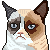dark-beam|Student Artist
Puzzling and superb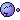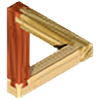Thanks. Btw, I am looking forward to tomorrow's thoughtful critique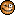dark-beam|Student Artist
Lool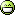this is more absurd than an impossible cubeLuca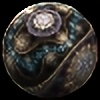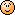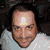one-tough-one|Hobbyist Digital Artist
Up, down, the in, the out, the upside down, the downside up take this string with you maze.Easy to get lost indeed, so no harm in bringing a long string. Better than breadcrumbs...Thanks. The triangle in the palette was a last minute idea, but a fun one I thought.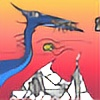timemit|Student Digital Artist
Real nice.. . I like the patterning in the code.. is that represented in the fractal?Thanks.

The pattern in the code has no effect actually. Due to the way that this fractal is constructed, it only requires a few colors from the 256 that are present in the palette. As the majority of colors is not used, I took that opportunity to make a pretty picture.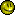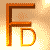Like it! I think it's an improvement!Thanks! Given that this was my first time using Apophysis, it took quite a bit of effort, so I'm glad to hear it was not in vain.Thanks. Yes, it may indeed look good on canvas. I am considering getting it printed on that myself.Join the community to add your comment. Already a deviant? Sign In
©2019 DeviantArt
All Rights reserved